Open in App
Not now

# Types of Friction

• Difficulty Level : Medium
• Last Updated : 09 Feb, 2021

Friction is a force between two surfaces that are sliding, or trying to slide, across each other. For example, when you try to push a book along the floor, friction makes this difficult. Friction always works in the direction opposite to the direction in which the object is moving, or trying to move. Friction always slows a moving object down.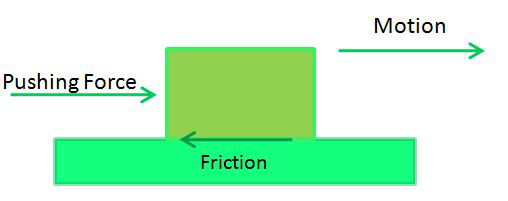The amount of friction depends on the materials from which the two surfaces are made. The rougher the surface, the more friction is produced. Friction also produces heat. If you rub your hands together quickly, you will feel them get warmer.

• Friction is responsible for many types of motion
• It helps us walk on the ground
• Brakes in a car make use of friction to stop the car
• Asteroids are burnt in the atmosphere before reaching Earth due to friction.
• It helps in the generation of heat when we rub our hands.

• Friction produces unnecessary heat leading to the wastage of energy.
• The force of friction acts in the opposite direction of motion, so friction slows down the motion of moving objects.
• Forest fires are caused due to the friction between tree branches.
• A lot of money goes into preventing friction and the usual wear and tear caused by it by using techniques like greasing and oiling.

## Types of Friction

There are basically four types of friction:

1. Static Friction
2. Sliding Friction
3. Rolling Friction
4. Fluid Friction

### Static Friction

It acts on objects when they are resting on a surface. For example, if you are hiking in the woods, there is static friction between your shoes and the trail each time you put down your foot. Without this static friction, your feet would slip out from under you, making it difficult to walk. In fact, that’s exactly what happens if you try to walk on ice. That’s because ice is very slippery and offers very little friction.

### Sliding Friction

It is the friction that acts on objects when they are sliding over a surface. Sliding friction is weaker than static friction. That’s why it’s easier to slide a piece of furniture over the floor after you start it moving than it is to get it moving in the first place. For example, There is sliding friction between the brake pads and bike rims each time you use your bike’s brakes. This friction slows the rolling wheels so you can stop.

### Rolling Friction

It is the friction that acts on objects when they are rolling over a surface. It is much weaker than sliding friction or static friction. This explains why most forms of ground transportation use wheels, including bicycles, cars, 4-wheelers, roller skates, scooters, and skateboards. For example: The ball bearings in this wheel reduce friction between the inner and outer cylinders when they turn.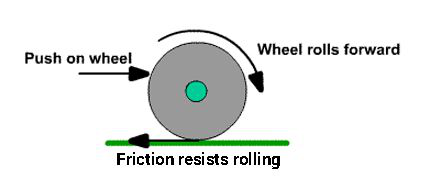### Fluid Friction

Fluid friction is friction that acts on objects that are moving through a fluid. A fluid is a substance that can flow and take the shape of its container. Fluids include liquids and gases. If you’ve ever tried to push your open hand through the water in a tub or pool, then you’ve experienced fluid friction. Another example could be a swimmer, cutting through the edges of water where the water provides fluid resistance to the swimmer.

## Coefficient of Friction

The coefficient of friction, µ, is a measure of the amount of friction existing between two surfaces. A low value of the coefficient of friction indicates that the force required for sliding to occur is less than the force required when the coefficient of friction is high. The value of the coefficient of friction is given byWhere,

F is the frictional force and,

N is the normal force.

### Derivation for coefficient of friction

Perpendicular force reduced

When an object is placed on an incline, the force perpendicular between the surfaces is reduced, according to the angle of the incline. The force required to overcome friction (Fr) equals the coefficient of friction (μ) times the cosine of the incline angle (cos θ) times the weight of the object (W). There are mathematical tables that give the values of cosines for various angles.

Fr = μ W Cosθ

Gravity contributes to sliding

Note that when an object is on an incline, the force of gravity contributes to causing the object to slide down the ramp or incline. Let’s call that force (Fg), and it is equal to the weight of the object (W) times the sine of the angle (sin θ)

Fg = W sin θ

Tangent of angle determines coefficient

If you put the ramp at a steep enough angle, Fg will become greater than Fr and the object will slide down the incline. The angle at which it just starts to slide is determined from the equation:

μ W cos θ = W sin θ

Dividing both sides of the equation by W and cos θ, we get the equation for the static coefficient of friction

μ = tan θ

where tan θ is the tangent of angle θ and equals.

For example: If you put a book on a ramp and changed the angle of the ramp until the book started to slide and then measured the angle of the ramp, you could determine the coefficient of friction between the book and the ramp. If the angle was 30 degrees, then the tangent of 30 degrees is about 0.58. That would be the static coefficient of friction in this case. Even if you increased the weight on the book, it would still slide at 30 degrees.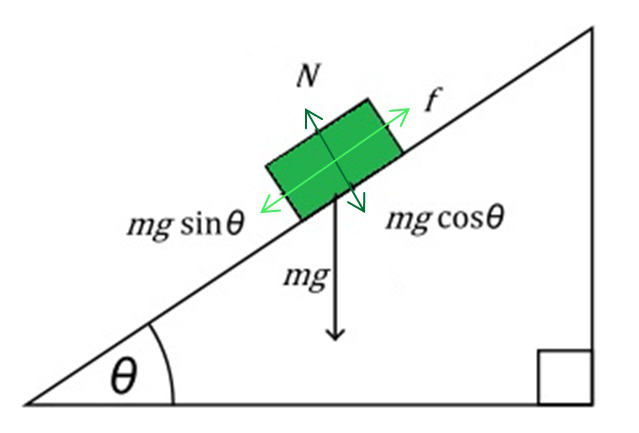### Sample Problems on Coefficient of Friction

Question 1: An object having a mass of 10 kg is placed on a smooth surface. Static friction between these two surfaces is given as the 15 N. Find the coefficient of static friction?

Solution:

Given

m = 10 kg

F = 30 N

We know that,

Normal force, N = mg

So, N = 10× 9.81 = 98.1 N

The formula for coefficient of static friction is,

μ = F/N

μ = 30/98.1

μ = 0.305

Question 2: The normal force and the frictional force of an object are 50 N and 80 N respectively. Find the coefficient of friction?

Solution:

Given

N = 50 N

F = 80 N

The formula for coefficient of friction is

μ = F/N

μ = 80/50

μ = 1.6 N

Question 3: A particle of mass 5 kg is at limiting equilibrium on a rough plane which is inclined at an angle of 30 degrees to the horizontal. Find the coefficient of friction between the particle and the plane.

Solution:

Resolving up the plane:Resolving perpendicular to the plane:In limiting equilibrium, so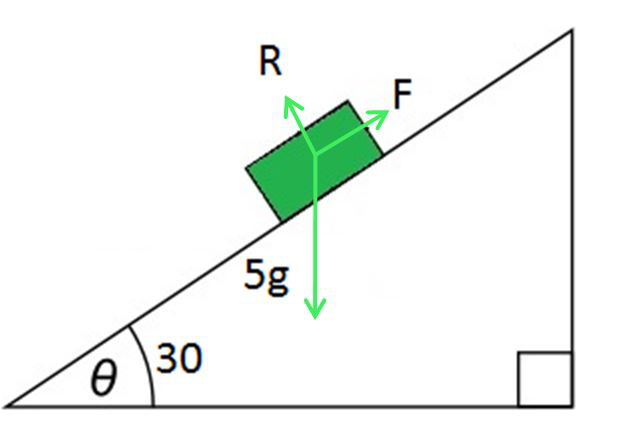Question 4: A 5 kg box on a horizontal table is pushed by a horizontal force of 15 N as shown on the right. If the coefficient of friction is 0.4, will the box move?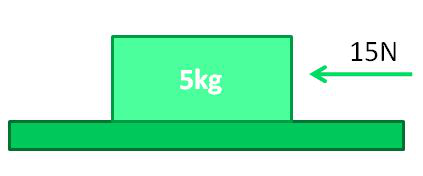Solution:

The sketch shows the forces acting on the box. Note that the weight of a box of mass 5 kg is 5g where

Since the vertical forces are in equilibrium,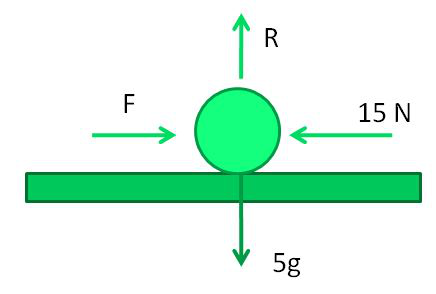Hence, the maximum possible friction is

The pushing force, 15 N, is less than this and so cannot overcome the friction.

The box will not move.

My Personal Notes arrow_drop_up